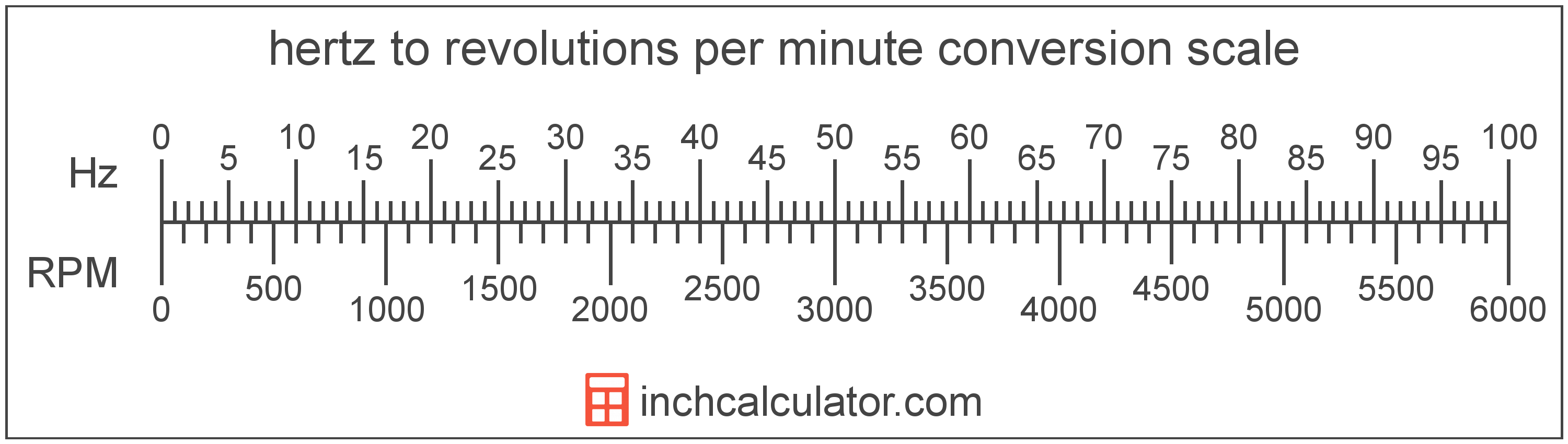# Hertz to Revolutions per Minute Converter

Enter the frequency in hertz below to get the value converted to revolutions per minute.

Results in Revolutions per Minute:1 Hz = 60 RPM

Do you want to convert revolutions per minute to hertz?

## How to Convert Hertz to Revolutions per Minute

To convert a measurement in hertz to a measurement in revolutions per minute, multiply the frequency by the following conversion ratio: 60 revolutions per minute/hertz.

Since one hertz is equal to 60 revolutions per minute, you can use this simple formula to convert:

revolutions per minute = hertz × 60

The frequency in revolutions per minute is equal to the frequency in hertz multiplied by 60.

For example, here's how to convert 5 hertz to revolutions per minute using the formula above.
revolutions per minute = (5 Hz × 60) = 300 RPM### How Many Revolutions per Minute Are in a Hertz?

There are 60 revolutions per minute in a hertz, which is why we use this value in the formula above.

1 Hz = 60 RPM

## What is a Hertz?

One hertz is formally defined as the frequency of one cycle per second.

The hertz is the SI derived unit for frequency in the metric system. Hertz can be abbreviated as Hz; for example, 1 hertz can be written as 1 Hz.

Frequency in hertz can be expressed using the formula:

Hz = Cycles / Time in Seconds

## What Are Revolutions per Minute?

Revolutions per minute are a measure of the number of rotations, turns, or revolutions, that an object makes each minute.

Revolutions per minute can be abbreviated as RPM, and are also sometimes abbreviated as rev/min or r/min. For example, 1 revolution per minute can be written as 1 RPM, 1 rev/min, or 1 r/min.

Revolutions per minute can be expressed using the formula:

RPM = Revolutions / Time in Minutes

## Hertz to Revolution per Minute Conversion Table

Table showing various hertz measurements converted to revolutions per minute.
Hertz Revolutions Per Minute
1 Hz 60 RPM
2 Hz 120 RPM
3 Hz 180 RPM
4 Hz 240 RPM
5 Hz 300 RPM
6 Hz 360 RPM
7 Hz 420 RPM
8 Hz 480 RPM
9 Hz 540 RPM
10 Hz 600 RPM
11 Hz 660 RPM
12 Hz 720 RPM
13 Hz 780 RPM
14 Hz 840 RPM
15 Hz 900 RPM
16 Hz 960 RPM
17 Hz 1,020 RPM
18 Hz 1,080 RPM
19 Hz 1,140 RPM
20 Hz 1,200 RPM
21 Hz 1,260 RPM
22 Hz 1,320 RPM
23 Hz 1,380 RPM
24 Hz 1,440 RPM
25 Hz 1,500 RPM
26 Hz 1,560 RPM
27 Hz 1,620 RPM
28 Hz 1,680 RPM
29 Hz 1,740 RPM
30 Hz 1,800 RPM
31 Hz 1,860 RPM
32 Hz 1,920 RPM
33 Hz 1,980 RPM
34 Hz 2,040 RPM
35 Hz 2,100 RPM
36 Hz 2,160 RPM
37 Hz 2,220 RPM
38 Hz 2,280 RPM
39 Hz 2,340 RPM
40 Hz 2,400 RPM

## References

1. National Institute of Standards and Technology, SI Units - Time, https://www.nist.gov/pml/owm/si-units-time